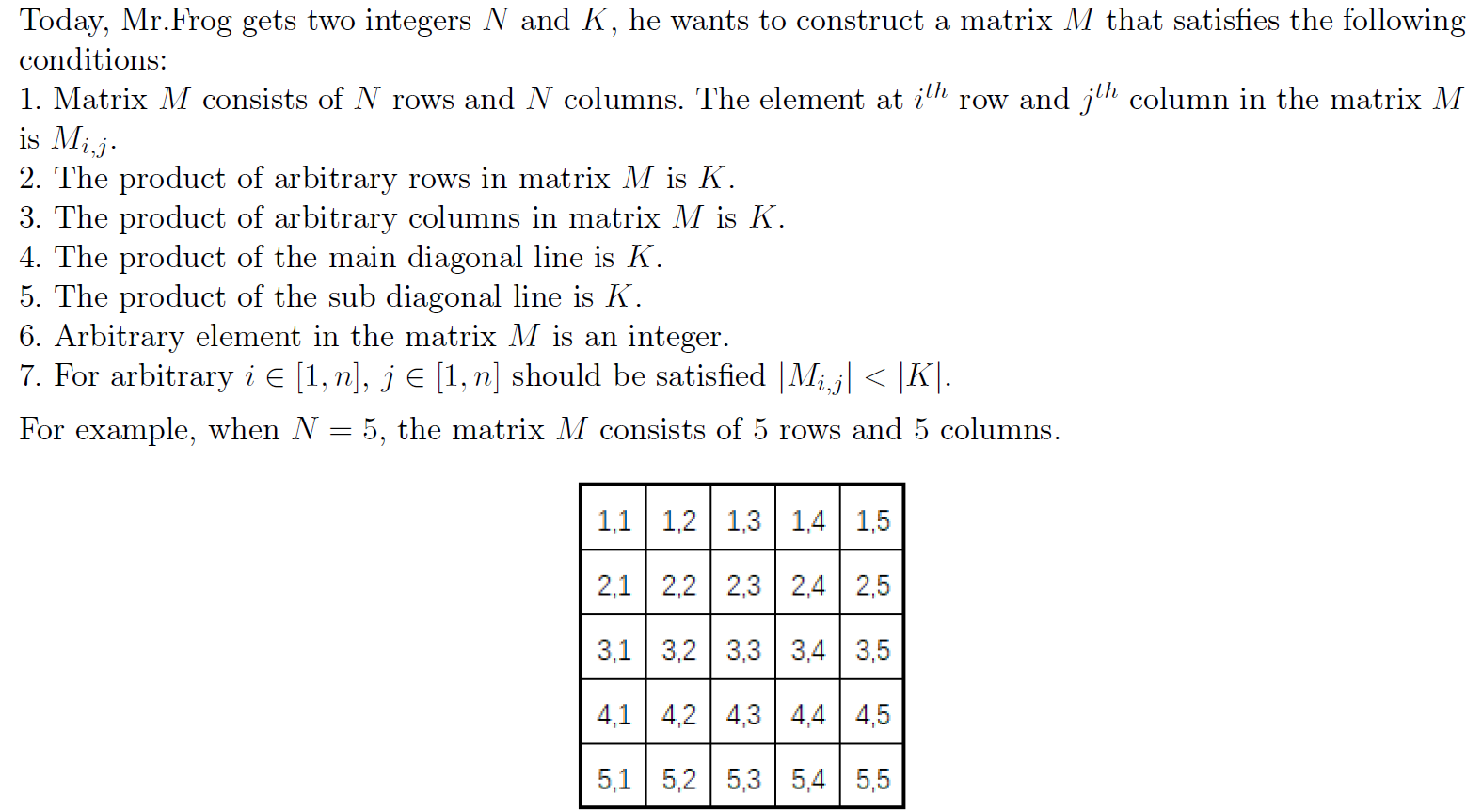odd doctor 已更新

# Limitless Matrix

Time Limit
30s
Memory Limit
32768KB
Judge Program
Standard
Ratio(Solve/Submit)
0.00%(0/16)
Description:The ith row consists of Mi,1, Mi,2, Mi,3, Mi,4 and Mi,5.
The jth column consists of M1,j , M2,j , M3,j , M4,j and M5,j .
The main diagonal line consists of M1,1, M2,2, M3,3, M4,4 and M5,5.
The sub diagonal line consists of M1,5, M2,4, M3,3, M4,2 and M5,1.
However, Mr.Frog will go to Hong Kong today. He asks you for help.

Input:

The first line contains an integer T, where T is the number of test cases. T test cases follow.

For each test case, the only line contains two integers N and K.

1<=T<=10

1<=N<=103

-109<=K<=109

Output:

For each test case, output one line containing “Case #x:”, where x is the test case number (starting from
1). If there is no solution, print “IMPOSSIBLE” in the next line; otherwise, print N lines with N integers.
If there are many multiple optimal answers, output any one of them.

Sample Input:
2
5 -7
3 373248
Sample Output:
Case #1:
IMPOSSIBLE
Case #2:
36 108 96
192 72 27
54 48 144

Submit